# isStateValid

Check if state is valid

## Syntax

``isValid = isStateValid(validatorObj,states)``

## Description

example

````isValid = isStateValid(validatorObj,states)` verifies if a set of given states are valid.```

## Examples

collapse all

This example shows how to validate paths through an evironment.

Load example maps. Use the simple map to create a binary occupancy map.

```load exampleMaps.mat map = occupancyMap(simpleMap); show(map)```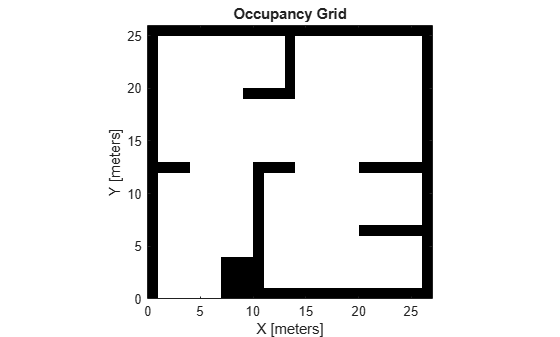Specify a coarse path through the map.

```path = [2 2 pi/2; 10 15 0; 17 8 -pi/2]; hold on plot(path(:,1),path(:,2),"--o")```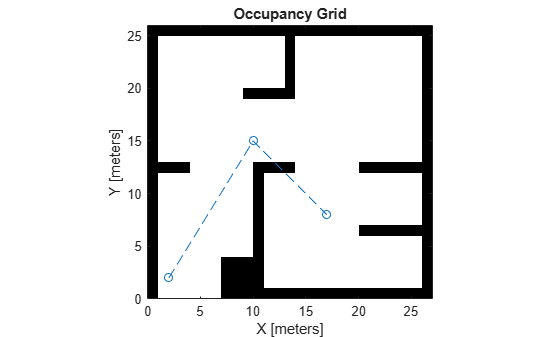Create a state validator using the `stateSpaceSE2` definition. Specify the map and the distance for interpolating and validating path segments.

```validator = validatorOccupancyMap(stateSpaceSE2); validator.Map = map; validator.ValidationDistance = 0.1;```

Check the points of the path are valid states. All three points are in free space, so are considered valid.

`isValid = isStateValid(validator,path)`
```isValid = 3x1 logical array 1 1 1 ```

Check the motion between each sequential path states. The `isMotionValid` function interpolates along the path between states. If a path segment is invalid, plot the last valid point along the path.

```startStates = [path(1,:);path(2,:)]; endStates = [path(2,:);path(3,:)]; for i = 1:2 [isPathValid, lastValid] = isMotionValid(validator,startStates(i,:),endStates(i,:)); if ~isPathValid plot(lastValid(1),lastValid(2),'or') end end hold off```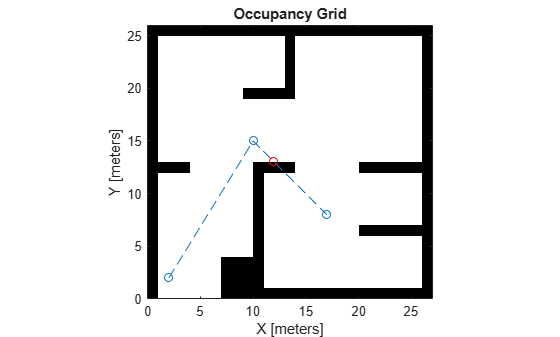This example shows how to validate paths through an evironment.

Load example maps. Use the simple map to create a vehicle cost map. Specify an inflation raidus of 1 meter.

```load exampleMaps.mat map = vehicleCostmap(double(simpleMap)); map.CollisionChecker = inflationCollisionChecker("InflationRadius",1); plot(map)```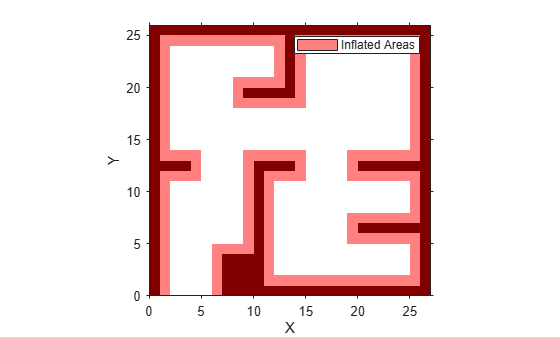Specify a coarse path through the map.

```path = [3 3 pi/2; 8 15 0; 17 8 -pi/2]; hold on plot(path(:,1),path(:,2),"--o")```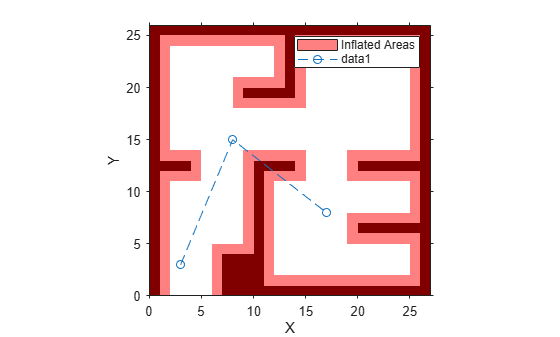Create a state validator using the `stateSpaceSE2` definition. Specify the map and the distance for interpolating and validating path segments.

```validator = validatorVehicleCostmap(stateSpaceSE2); validator.Map = map; validator.ValidationDistance = 0.1;```

Check the points of the path are valid states. All three points are in free space, so are considered valid.

`isValid = isStateValid(validator,path)`
```isValid = 3x1 logical array 1 1 1 ```

Check the motion between each sequential path states. The `isMotionValid` function interpolates along the path between states. If a path segment is invalid, plot the last valid point along the path.

```startStates = [path(1,:);path(2,:)]; endStates = [path(2,:);path(3,:)]; for i = 1:2 [isPathValid, lastValid] = isMotionValid(validator,startStates(i,:),endStates(i,:)); if ~isPathValid plot(lastValid(1),lastValid(2),'or') end end hold off```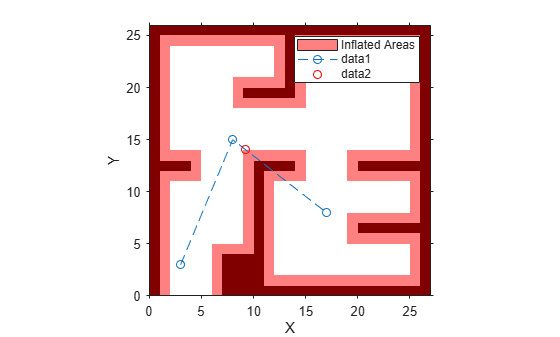## Input Arguments

collapse all

State validator object, specified as an object of subclass of `nav.StateValidator`. For provided state validator objects, see `validatorOccupancyMap` or `validatorVehicleCostmap`.

Initial state position, specified as a n-element vector or m-by-n matrix of row vectors. n is the dimension of the state space specified in `validatorObj`. m is the number of states to validate.

## Output Arguments

collapse all

Valid states, specified as a m-element vector of `1`s and `0`s.